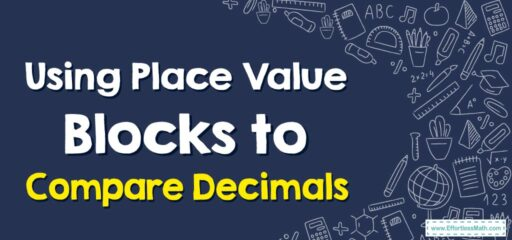# How to Use Place Value Blocks to Compare Decimals

Place value blocks, also known as base-10 blocks, can be used to compare decimals visually. These manipulatives come in various sizes to represent ones (or units), tenths, hundredths, and thousandths.## A Step-by-step Guide to Using Place Value Blocks to Compare Decimals

Using place value blocks is an effective way to visually compare decimals. Here’s a step-by-step guide on how to use place value blocks to compare decimals:

### Step 1: Identify the decimals:

Write down the decimal numbers you want to compare.

### Step 2: Gather place value blocks:

Obtain a set of place value blocks or base-10 blocks that represent ones (or units), tenths, hundredths, and thousandths:

• A large cube represents one whole (1 or one unit).
• A flat represents one-tenth (0.1).
• A long (or rod) represents one-hundredth (0.01).
• A small cube (or unit) represents one-thousandth (0.001).

### Step 3: Represent the decimals using place value blocks:

For each decimal number, use the appropriate place value blocks to represent the value. Arrange the blocks for each decimal number in separate groups.

### Step 4: Compare the decimals visually:

Lay out the place value blocks for each decimal number side by side or one above the other, aligning them by their place values (ones, tenths, hundredths, and thousandths).

### Step 5: Analyze the place value blocks:

Compare the blocks at each place value, starting with the largest place value (usually ones) and moving to the right. Determine which decimal is larger or smaller based on the number of blocks in each place value.

### What people say about "How to Use Place Value Blocks to Compare Decimals - Effortless Math: We Help Students Learn to LOVE Mathematics"?

No one replied yet.

X
30% OFF

Limited time only!

Save Over 30%

SAVE $5 It was$16.99 now it is \$11.99